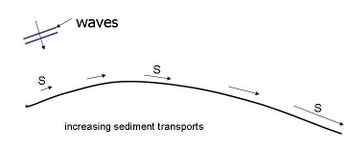# Example: Erosion because of a convex coastFigure 1 Convex piece of coast Erosion because of a convex coast Consider a part of a sandy coast, a few kilometres long. In plan view it refers to a convex coast (see Figure 1). In the figure only the waterline is indicated, but it is assumed that the depth contours are more or less parallel to the waterline. Waves approach the coast as indicated in the figure. Along the part of the coast as has been plotted, the angle between the wave crests and the orientation of the coast is ever changing. Longshore currents and longshore sediment transports [[math]S[/math]: e.g. in m3/year] are generated. In Figure 1 the magnitude and direction of the longshore sediment transport rates are schematically indicated. Gradients in the longshore sediment transport rates seem to occur [[math]dS/dx[/math] is not equal to 0 in m3/m.year]. Due to the gradients loss of sediments out of the control volume area (occur; volume [math]V[/math] (m3/m) is diminishing [[math]dV/dt = dS/dx[/math]]. See for the definition of control volume Figure 1 in article types and background of coastal erosion